# Practice problems

## Indifference curves and budget lines

Imagine that someone needs to decide how to spend their entire budget on some combination of waffles and calzones (to stick with the Parks and Rec theme). The three problems below show three different sets of budgets, prices, and utility functions. Help each person maximize their utility by finding the indifference curve that is tangent to the budget line.

### Practice problem 1

Given this background information…

• Waffles ($$x$$): $5 • Calzones ($$y$$):$6
• Budget: $80 • Utility: $$u = x^2 \ 3y$$ • Marginal utility (or MRS, or first derivative of utility): $$\frac{2y}{x}$$ …calculate the following: • The optimal quantity of waffles • The optimal quantity of calzones • The utility generated from this level of consumption ### Practice problem 2 Given this background information… • Waffles ($$x$$):$3.50
• Calzones ($$y$$): $8 • Budget:$120
• Utility: $$u = \sqrt{2x \ 8y}$$
• Marginal utility (or MRS, or first derivative of utility): $$\frac{y}{x}$$

…calculate the following:

• The optimal quantity of waffles
• The optimal quantity of calzones
• The utility generated from this level of consumption

### Practice problem 3

Given this background information…

• Waffles ($$x$$): $4 • Calzones ($$y$$):$10
• Budget: 200 • Utility: $$u = 0.4x \times y^2$$ • Marginal utility (or MRS, or first derivative of utility): $$\frac{y}{2x}$$ …calculate the following: • The optimal quantity of waffles • The optimal quantity of calzones • The utility generated from this level of consumption ## Supply, demand, taxes, and deadweight loss ### Practice problem 1 Imagine a market where the demand and supply curves are defined with the following formulas: \begin{aligned} \text{Demand:}& & P = 15 - 2Q \\ \text{Supply:}& & P = 3 + 0.5Q \end{aligned} The government imposes a5 tax on suppliers.

1. What is the pre-tax equilibrium price and quantity?
2. What is the pre-tax consumer and producer surplus?
3. What is the post-tax price and quantity?
4. What is the post-tax consumer and producer surplus?
5. How much deadweight loss is incurred?
6. How much tax revenue is generated?
7. What percentage of the tax burden is borne by consumers and producers?

### Practice problem 2

Imagine a market where the demand and supply curves are defined with the following formulas:

\begin{aligned} \text{Demand:}& & P = 133 - 1.067Q \\ \text{Supply:}& & P = 14 + 0.75Q \end{aligned}

The government imposes a 48 tax on suppliers. Answer these questions: 1. What is the pre-tax equilibrium price and quantity? 2. What is the pre-tax consumer and producer surplus? 3. What is the post-tax price and quantity? 4. What is the post-tax consumer and producer surplus? 5. How much deadweight loss is incurred? 6. How much tax revenue is generated? 7. What percentage of the tax burden is borne by consumers and producers? ### Practice problem 3 Imagine a market where the demand and supply curves are defined with the following formulas: \begin{aligned} \text{Demand:}& & P = 592 - 2.852Q \\ \text{Supply:}& & P = 28 + 0.2Q \end{aligned} The government imposes a56 tax on suppliers.

1. What is the pre-tax equilibrium price and quantity?
2. What is the pre-tax consumer and producer surplus?
3. What is the post-tax price and quantity?
4. What is the post-tax consumer and producer surplus?
5. How much deadweight loss is incurred?
6. How much tax revenue is generated?
7. What percentage of the tax burden is borne by consumers and producers?

### Utility maximization 1

• Optimal number of waffles: 10.667
• Optimal number of calzones: 4.444
• Utility: 1517.04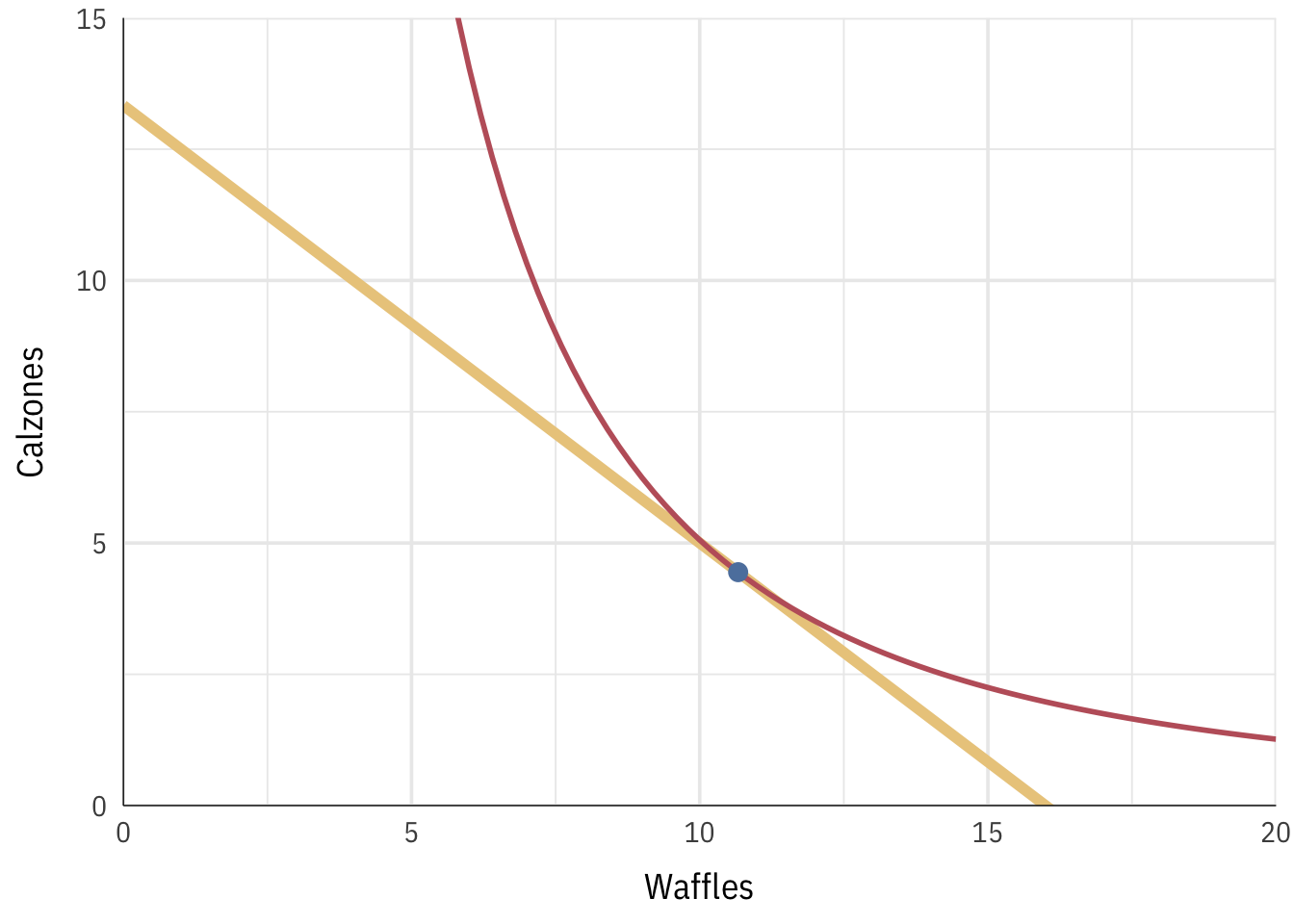### Utility maximization 2

• Optimal number of waffles: 17.143
• Optimal number of calzones: 7.5
• Utility: 45.36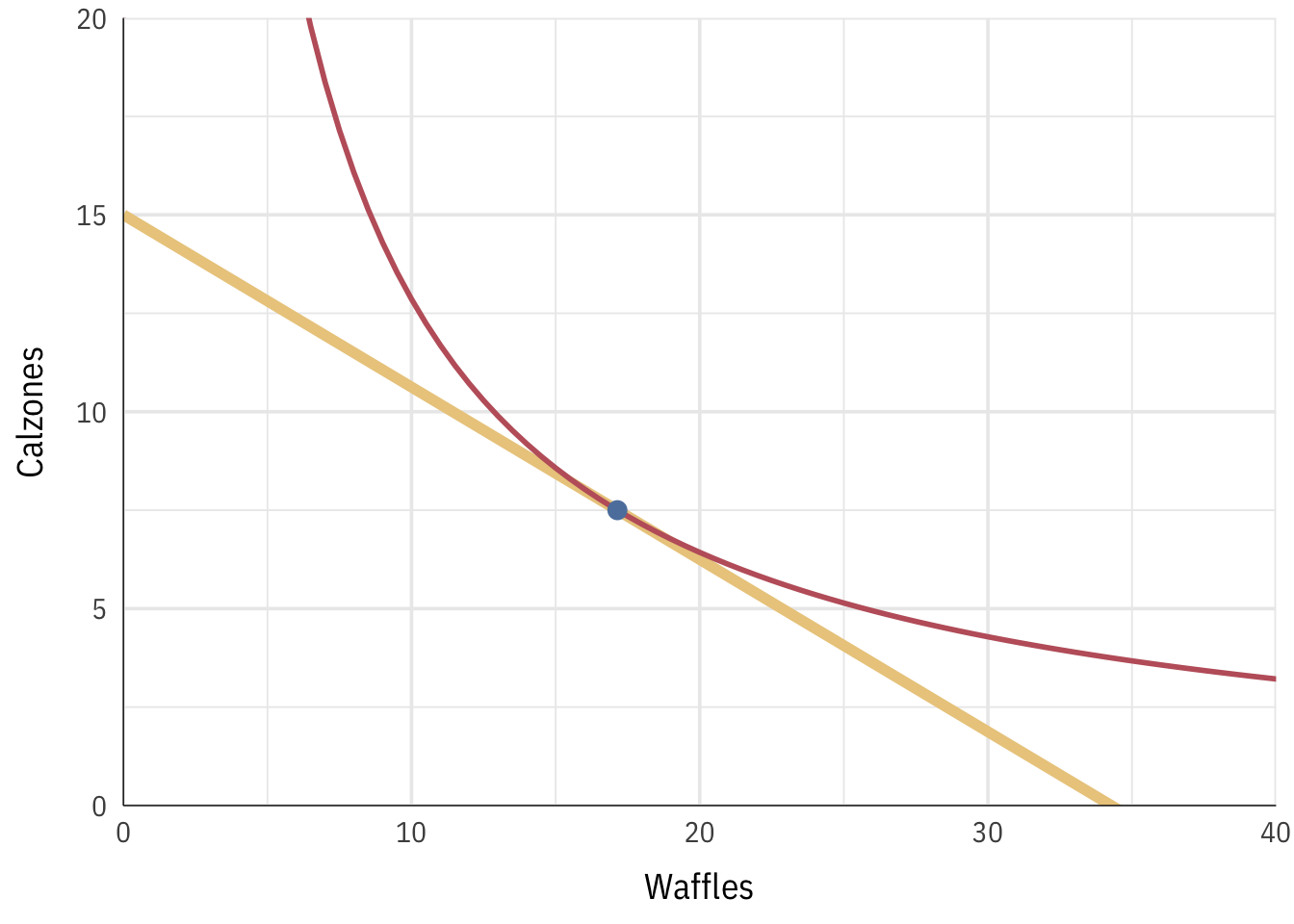### Utility maximization 3

• Optimal number of waffles: 16.667
• Optimal number of calzones: 13.333
• Utility: 1185.19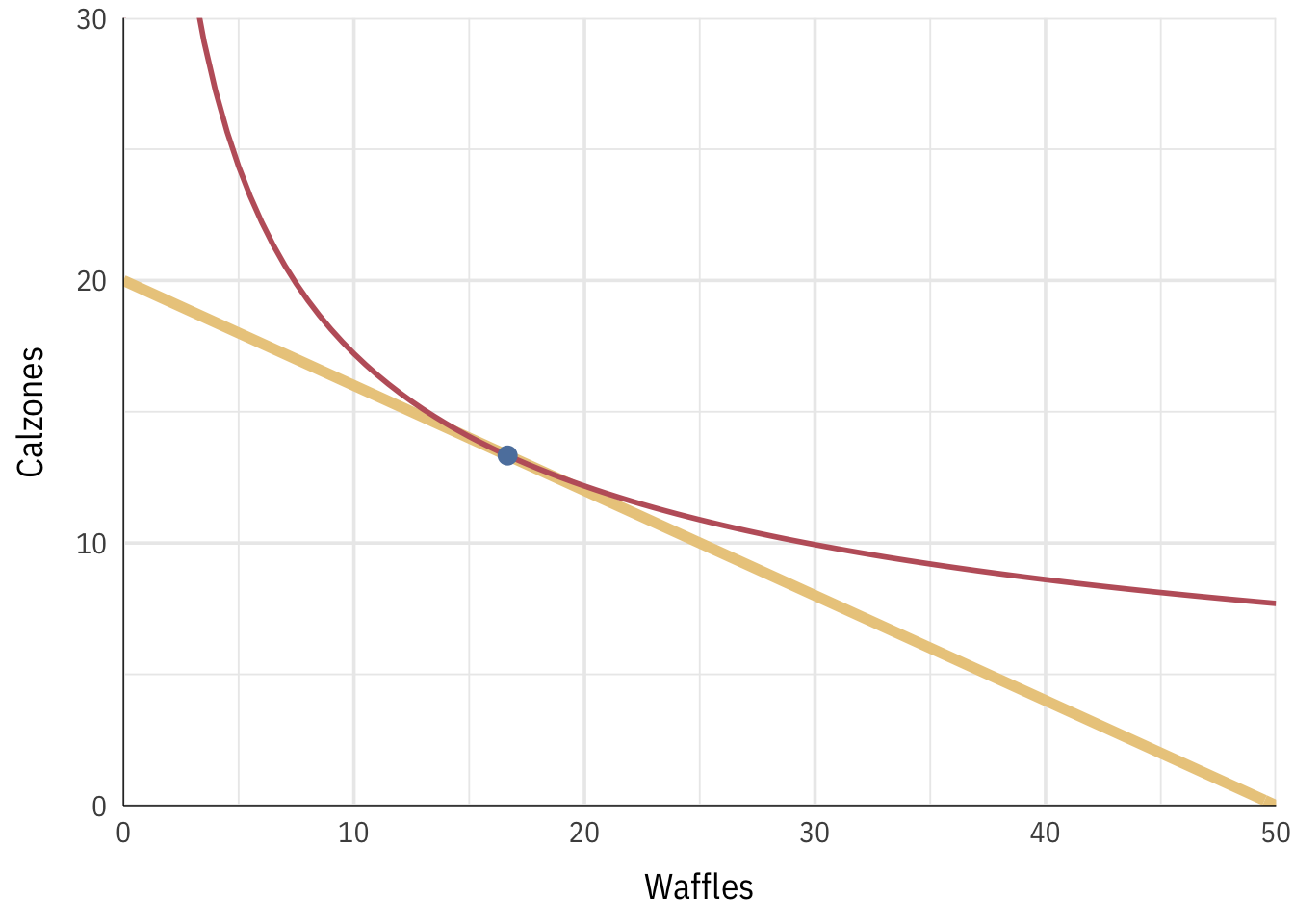### Supply and demand 1

Post-tax supply:

$P = 8 + 0.5Q$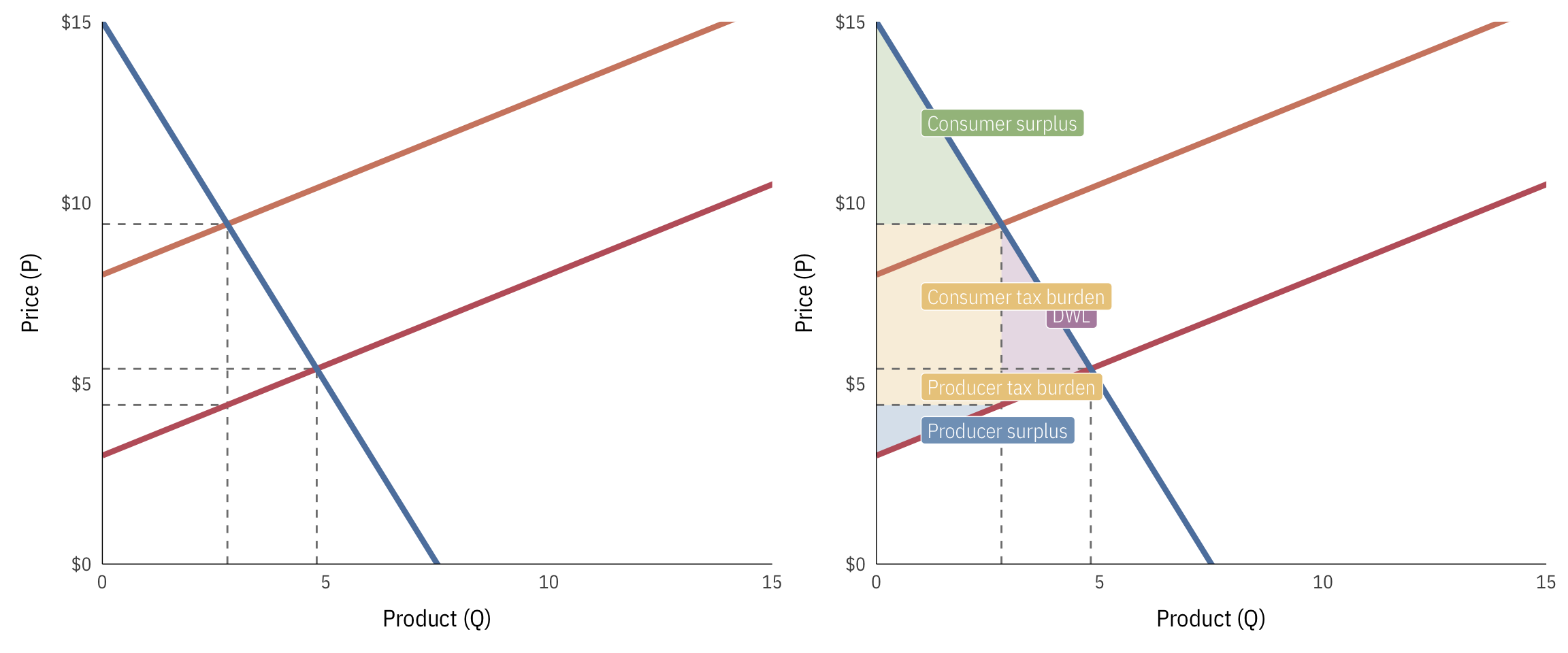• Pre-tax quantity: 4.8
• Pre-tax price: $5.4 • Pre-tax consumer surplus:$23.04 ($$1/2 \times 4.8 \times 9.6$$)
• Pre-tax producer surplus: $5.76 ($$1/2 \times 4.8 \times 2.4$$) • Post-tax quantity: 2.8 • Post-tax price:$9.4
• Post-tax consumer surplus: $7.84 ($$1/2 \times 2.8 \times 5.6$$) • Post-tax producer surplus:$1.96 ($$1/2 \times 2.8 \times 1.4$$)
• Deadweight loss: $5 ($$1/2 \times 2 \times 5$$) • Total tax incidence (revenue raised):$14 ($$(9.4 - 4.4) \times 2.8$$)
• Consumer tax incidence: $11.2 ($$(9.4 - 5.4) \times 2.8$$) • Producer tax incidence:$2.8 ($$(5.4 - 4.4) \times 2.8$$)
• Percent of tax borne by consumers: 80% ($$11.2 / 14$$)
• Percent of tax borne by producers: 20% ($$2.8 / 14$$)

### Supply and demand 2

Post-tax supply:

$P = 62 + 0.5Q$• Pre-tax quantity: 65.49
• Pre-tax price: $63.12 • Pre-tax consumer surplus:$2288.33 ($$1/2 \times 65.49 \times 69.88$$)
• Pre-tax producer surplus: $1608.48 ($$1/2 \times 65.49 \times 49.12$$) • Post-tax quantity: 39.08 • Post-tax price:$91.31
• Post-tax consumer surplus: $814.59 ($$1/2 \times 39.08 \times 41.69$$) • Post-tax producer surplus:$572.58 ($$1/2 \times 39.08 \times 29.31$$)
• Deadweight loss: $634.01 ($$1/2 \times 26.42 \times 48$$) • Total tax incidence (revenue raised):$1875.62 ($$(91.31 - 43.31) \times 39.08$$)
• Consumer tax incidence: $1101.42 ($$(91.31 - 63.12) \times 39.08$$) • Producer tax incidence:$774.2 ($$(63.12 - 43.31) \times 39.08$$)
• Percent of tax borne by consumers: 59% ($$1101.42 / 1875.62$$)
• Percent of tax borne by producers: 41% ($$774.2 / 1875.62$$)

### Supply and demand 3

Post-tax supply:

$P = 84 + 0.2Q$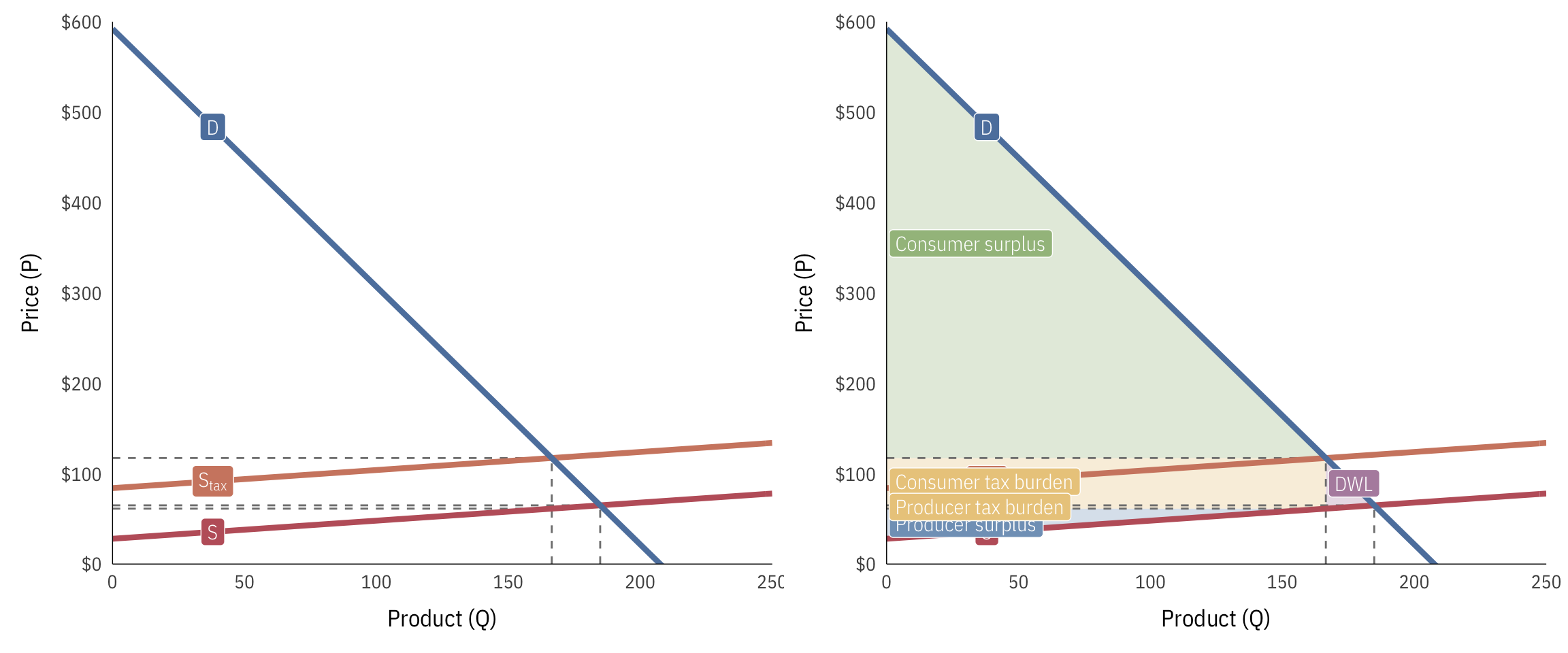• Pre-tax quantity: 184.8
• Pre-tax price: $64.96 • Pre-tax consumer surplus:$48697.73 ($$1/2 \times 184.8 \times 527.04$$)
• Pre-tax producer surplus: $3414.99 ($$1/2 \times 184.8 \times 36.96$$) • Post-tax quantity: 166.45 • Post-tax price:$117.29
• Post-tax consumer surplus: $39507.35 ($$1/2 \times 166.45 \times 474.71$$) • Post-tax producer surplus:$2770.5 ($$1/2 \times 166.45 \times 33.29$$)
• Deadweight loss: $513.76 ($$1/2 \times 18.35 \times 56$$) • Total tax incidence (revenue raised):$9321.1 ($$(117.29 - 61.29) \times 166.45$$)
• Consumer tax incidence: $8710.28 ($$(117.29 - 64.96) \times 166.45$$) • Producer tax incidence:$610.82 ($$(64.96 - 61.29) \times 166.45$$)
• Percent of tax borne by consumers: 93% ($$8710.28 / 9321.1$$)
• Percent of tax borne by producers: 7% ($$610.82 / 9321.1$$)